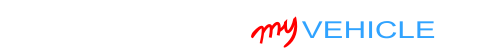## Interpreting Your Compression Test Results

A low compression value in one or more cylinders can cause a misfire problem or a rough idle problem -depending on how low the value is.

To be a bit more specific, a low compression value doesn't necessarily mean that that cylinder is causing a problem.

It's when the low compression value is lower than 15% of the highest one, that that cylinder will cause a misfire problem or a rough idle problem.

The cool thing is that we can easily find out if the low compression values are causing an engine performance problem or not.

To find out, we need to figure out if the low compression values are lower than 15% of the highest compression value you got.

You can do this (figuring out the 15%) in one of two ways: You can calculate this 15% difference with pen and paper or you can use my low compression calculator. You can find the low compression calculator here: Online Low Engine Compression Calculator (at: easyautodiagnostics.com).

If you want to manually calculate the 15% difference, here's what you'll need to do:

1. STEP 1: Multiply the highest compression value by 0.15 (this is the decimal value of 15%).
2. STEP 2: Round the result to the nearest one (for example: 25.6 would become 26).
3. STEP 3: Subtract the result (the number that was rounded) from the highest compression value.
4. ANSWER: The result of this subtraction is the lowest possible compression value any cylinder can have.

Now, let me give you a more specific example: Let's say that I got the following compression readings:

Cylinder Pressure
#1 165 PSI
#2   95 PSI
#3 155 PSI
#4 175 PSI

My next step is to do the following calculation:

1. STEP 1:  175 x 0.15 = 26.25.
2. STEP 2:  26.25 = 26 (rounded to nearest one).
3. STEP 3:  175 - 26 = 149.
4. ANSWER:  149 PSI. Any cylinder with this compression (or lower) value will misfire.

Since cylinder #2 is only producing 95 PSI, I can now conclude that it's 'dead' and causing a misfire.

To find out if the lowest compression value you got from your engine compression test is within a good range, you'll need to do the same calculation. Of course, you'll need to use the highest compression value you got and not the one in the example.

Once you've found the 'dead' cylinder, the next step is to find out what's causing the low compression value. For this step, go to: TEST 2: ‘Wet’ Engine Compression Test.

## TEST 2: ‘Wet’ Engine Compression TestIn this test step you're gonna' add about two tablespoons of oil to the engine cylinder that showed a low compression reading in the ‘Dry’ compression test.

The engine oil that you're gonna' add to the cylinder will help determine if the low cylinder pressure or pressures you recorded in the ‘Dry’ compression test are caused by worn piston rings or worn cylinder head valves.

Depending on whether the compression pressure rises (on your compression tester) or not, you'll be able to say that the problem lies in the piston's rings or in the cylinder head valves.

OK, this is what you need to do:

1. 1

Add a small amount of engine oil to the cylinder that reported low compression or no compression in the ‘Dry’ compression test.

The amount should be about 1 to 2 tablespoons of oil.

2. 2

Install the compression tester onto the cylinder.

Do not use any type of tool to tightened the compression tester. Hand tight is fine.

3. 3

When all is set up, have your helper crank the engine.

4. 4

You'll get one of two results:

1.) The compression value will go up (from the one you recorded before).

2.) The compression value will stay the same.

CASE 1: The compression value shot up. This tells you that the piston compression rings are worn out and thus the problem is in the bottom end (block) of the engine in your 1.6L Honda Civic.

CASE 2: The compression value stayed the same. This confirms that the problem is in the cylinder head valves.

## More 1.6L Honda Civic Tutorials

You can find all of the 1.6L Honda articles here: Honda 1.6L Index Of Articles.

Here's a sample of the tutorials you'll find in the index:If this info saved the day, buy me a beer!Honda Vehicles:

• Civic 1.6L
• 1995, 1996, 1997, 1998, 1999, 2000
• Civic del Sol 1.6L
• 1994, 1995, 1996, 1997